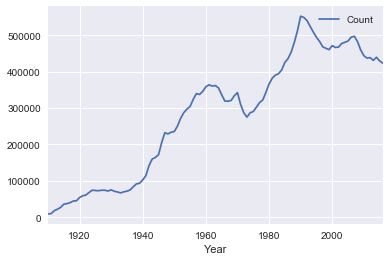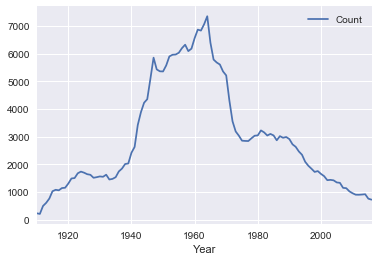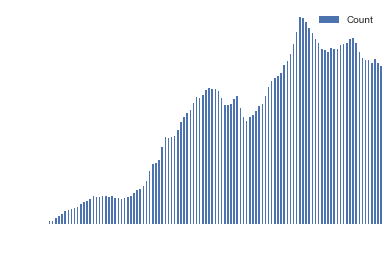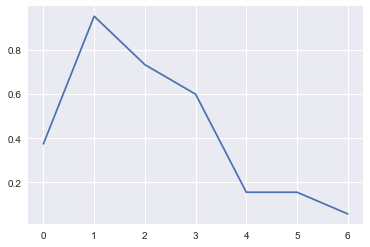# Python Libraries: Matplotlib and Seaborn Tutorial

## How to Use Matplotlib and Seaborn for DatascienceMatplotlib reference: https://matplotlib.org/2.1.2/index.html
Seaborn reference: https://seaborn.pydata.org/

Matplotlib is a ubiquitious plotting library for python with infinite customization. Seaborn allows you to make graphs very quickly and beautifully though with less modification options. Both are very compatible with pandas and numpy.

Install jupyter:

``````pip3 install jupyter
``````

Launch your notebook (opens in browser):

``````jupyter notebook [name_of_file.ipynb]
``````

Alternatively, you can run Jupyter Notebooks in Google Drive using Colaboratory.

## Import

Note: We’ll be relying on Pandas and Numpy in this tutorial.

We need to import `matplotlib`! Adding `%matplotlib inline` will make plotting a bit more convenient.

``````import numpy as np
import pandas as pd
import matplotlib.pyplot as plt
%matplotlib inline
``````

## Using Matplotlib built into Pandas

If you have a Pandas dataframe, it actually comes with some basic plotting functions that run the matplotlib code for you. It’s a nice shortcut!

`yearly_data` contains the number of registered babies per year.

``````yearly_data.head()
``````
Count
Year
1910 9164
1911 9984
1912 17944
1913 22094
1914 26925

## Line Graphs

``````yearly_data.plot(kind="line")  #kind='line' is optional
``````
``````<matplotlib.axes._subplots.AxesSubplot at 0x11a052198>
``````### Study: Name History

``````# don't worry about this function unless you want to learn about groupby
def your_name_history(name):
return baby_names[baby_names['Name'] == name].groupby('Year').sum()
``````
``````table = your_name_history('John')

table.plot()
``````
``````<matplotlib.axes._subplots.AxesSubplot at 0x114a44470>
``````## Bar Graphs

We can modify our data before we graph it to analyze different things.

``````yearly_data.plot(kind="bar")
plt.axis('off')
``````
``````(-0.5, 106.5, 0.0, 580000.05000000005)
``````Class Exercise:

How could we graph only the 15 years after World War II (i.e. 1945-1960)?

Hint: create a table with only the desired years first

``````modified = yearly_data.loc[1945:1960]

modified.plot(kind="bar", figsize=(15,8))
``````
``````<matplotlib.axes._subplots.AxesSubplot at 0x11b7f5fd0>
``````# Plot with Pandas

## Line Graphs

Use `plt.plot()` to create line graphs! The required arguments are a list of x-values and a list of y-values.

``````np.random.seed(42) # To ensure that the random number generation is always the same
plt.plot(np.arange(0, 7, 1), np.random.rand(7, 1))
plt.show()
````````````%matplotlib inline

plt.plot(np.arange(0, 7, 1), np.random.rand(7, 1))
# plt.show() no longer required
``````
``````[<matplotlib.lines.Line2D at 0x11bfb12e8>]
``````